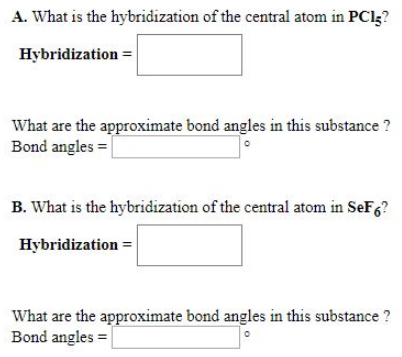# Problem: A. What is the hybridization of the central atom in PCl5? What are the approximate bond angles in this substance? B. What is the hybridization of the central atom in SeF6? What are the approximate bond angles in this substance?

###### FREE Expert Solution
82% (30 ratings)###### Problem Details

A. What is the hybridization of the central atom in PCl5

What are the approximate bond angles in this substance?

B. What is the hybridization of the central atom in SeF6

What are the approximate bond angles in this substance?